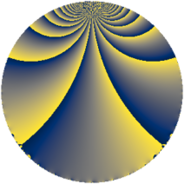# Properties

 Label 105.2.jLevel $105$ Weight $2$ Character orbit 105.j Rep. character $\chi_{105}(8,\cdot)$ Character field $\Q(\zeta_{4})$ Dimension $24$ Newform subspaces $1$ Sturm bound $32$ Trace bound $0$

# Related objects

## Defining parameters

 Level: $$N$$ $$=$$ $$105 = 3 \cdot 5 \cdot 7$$ Weight: $$k$$ $$=$$ $$2$$ Character orbit: $$[\chi]$$ $$=$$ 105.j (of order $$4$$ and degree $$2$$) Character conductor: $$\operatorname{cond}(\chi)$$ $$=$$ $$15$$ Character field: $$\Q(i)$$ Newform subspaces: $$1$$ Sturm bound: $$32$$ Trace bound: $$0$$

## Dimensions

The following table gives the dimensions of various subspaces of $$M_{2}(105, [\chi])$$.

Total New Old
Modular forms 40 24 16
Cusp forms 24 24 0
Eisenstein series 16 0 16

## Trace form

 $$24q - 4q^{3} + O(q^{10})$$ $$24q - 4q^{3} - 16q^{10} + 16q^{12} - 8q^{13} - 16q^{15} - 16q^{16} - 20q^{18} + 4q^{21} + 8q^{22} - 16q^{25} - 16q^{27} + 20q^{30} + 28q^{33} + 16q^{36} - 16q^{37} + 64q^{40} - 20q^{42} - 40q^{43} + 20q^{45} - 64q^{46} + 16q^{48} - 20q^{51} + 40q^{55} + 4q^{57} + 40q^{58} + 32q^{60} + 32q^{61} - 8q^{63} - 16q^{66} + 24q^{67} - 8q^{70} - 8q^{72} + 32q^{73} - 60q^{75} + 32q^{76} + 60q^{78} + 52q^{81} - 80q^{82} + 24q^{85} + 4q^{87} + 96q^{88} - 24q^{90} - 24q^{91} - 76q^{93} - 96q^{96} + 24q^{97} + O(q^{100})$$

## Decomposition of $$S_{2}^{\mathrm{new}}(105, [\chi])$$ into newform subspaces

Label Dim. $$A$$ Field CM Traces $q$-expansion
$$a_2$$ $$a_3$$ $$a_5$$ $$a_7$$
105.2.j.a $$24$$ $$0.838$$ None $$0$$ $$-4$$ $$0$$ $$0$$